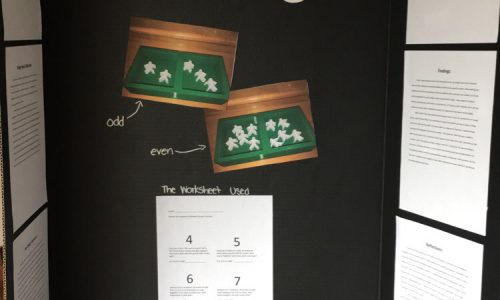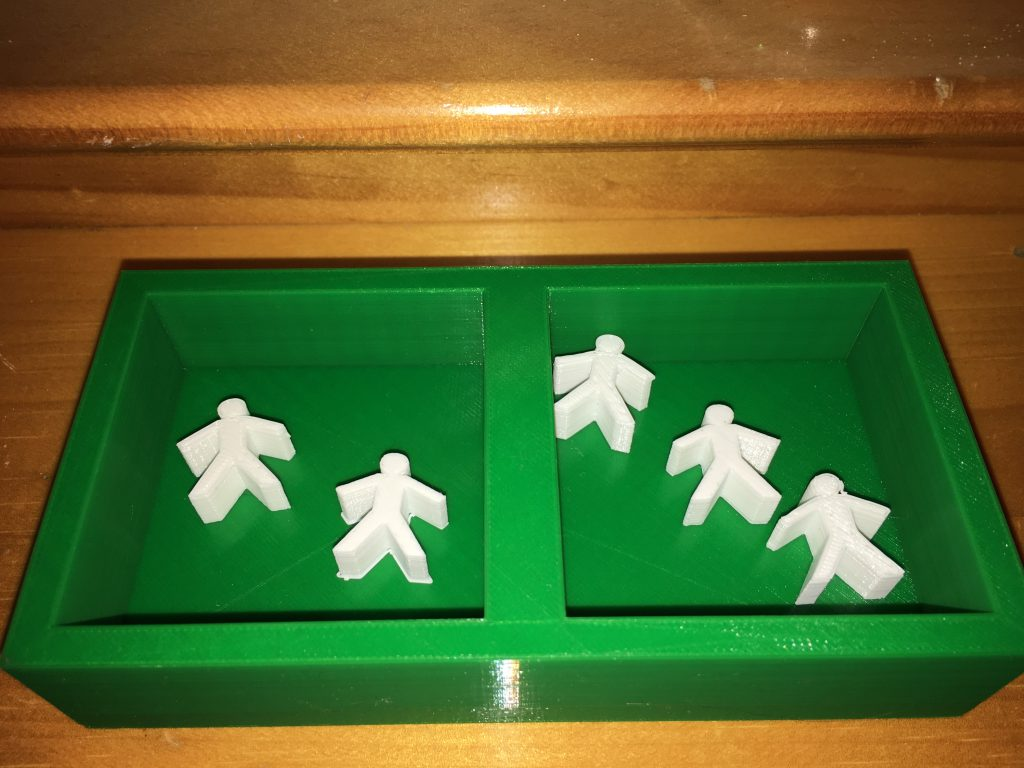# Even Number ToolFor my 3-D design project, I designed a manipulative that I believe will help students learn what an even number is. As we discussed, it is better to learn what something is and understand it, instead of simply memorizing the facts. Therefore, for my manipulative to help learn what even numbers are, I scratched my puzzle idea. In the end, I created a container and different edged prisms.

The purpose of the manipulative is to separate the pieces of each prism, one at a time, into the two sides of the container. An even number is characterized as, “Any integer (not a fraction) that can be divided exactly by 2” (Definition of an even number). Therefore, if both sides of the container have an equal number of edges, that means that the number can be divided equally into 2 parts, which makes the number even. I think this manipulative should work from a mathematical learning point of view, because the two parts of the container represent the two halves of the whole. When the two halves have the same amount of edges in them, it will show that the number of edges can be divided by two equally.

The prisms, when looking at them from above, have four to seven edges. The amount of edges per prism represents both even and odd numbers. This will help students really have to think and not assume that a number is even based on if the number of edges is a number included in this manipulative. Since the students cannot assume, they will have to think and try to understand why having two edges on each side makes the prism’s number of edges a number even.  Students will also have to try to understand why two edges of the prism on one side of the container and three edges of the prism on the other side will help prove that the number of edges to that particular prism is not an even number. This should help students learn what even numbers can be when working with the design I have created.

I feel that learning often happens through exploration and believe that children often learn and work better when they have manipulatives to use. Those beliefs, along with my understanding of math led to my design of the manipulative. I feel that there are enough prisms to explore with, for younger elementary school grades, and the container to go with it helps the students separate the pieces in a way that the pieces can be counted without necessarily putting them all back into a single pile. The design also allows for some flexibility. Students can count the number of pieces per container as they go along, or they can separate the pieces and then count each side after the edges have been placed into the two sides of the container.

In terms of learning, my project would be considered successful if students were able to use it as a manipulative or even as a math center. I hope the project could be used for students to explore and better learn what it means for a number to be considered an even number. The students would be able to explore and see what an even number is. This could even be used as in introduction to even numbers. For example, a teacher could give them to students and say which prisms have an even number of edges and which have an odd number of edges. From there, students would have to use their imagination, think critically, and fully involve themselves with the design to look for possibilities to why the even numbers are even and why the odd numbers are not even.

If the project was successful, it could even help teach the students how to tell if a number is odd. The students can learn that an odd number is not a number that equally divides by two. From the knowledge they learn, they can see the differences. Students could also come up with their own reasoning that the design allows them to think of for why an odd number is odd.

If my project failed to work, there would be at least one of two reasons behind the failure. The first reason of failure would be the reason for me not doing a puzzle. The project would fail if students memorized the rule for even numbers, instead of using the design to explore and learn. The second reason that the project would fail to work, would be if the students were unable to comprehend how to learn about even numbers from the design. Since this particular manipulative was designed, with the purpose of helping students learn what an even number is, the project would fail if students were not able to come to the conclusions or if students struggled using the manipulative in general.

In terms of testing my design, I have a few options.           My first option would be to see if the teacher I work for would allow me to test the project in the classroom of 5. The class is an LLD classroom for Grade 1 and Grade 2 students. The age group and capabilities in math would be appropriate for the project, and the students would help me see the project being tested out in a real-life, classroom setting.

My second option would be to reach out to my mother’s friends who teaches children in K-2 and have those children test out the design. I could see a few children at two different times based on locations in New Jersey (some live in North Jersey and some live in South Jersey). I could have one group use them knowing beforehand what makes an even number even and have the other group use the project not knowing what makes an even number even. This would show me if the project could be used both ways in a classroom.

If neither of the two are doable options, I can reach out to my father and step-mother. I have a half-sister who is in Grade 1, so she would also be an acceptable grade level to test out my project on. With my three options and option 2 having some flexibility, I know that I can test out the project without any issues.